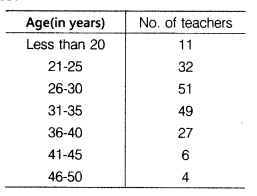# For a particular year, following is the distribution of the ages (in years) of primary

For a particular year, following is the distribution of the ages (in years) of primary school teachers in a particular state.(i)Determine the class limits of the fourth class.

(ii)What is the class size?
(iii)Construct a cumulative frequency table.
(iv)Find the modal class interval.

(i) Class limits of 4th class
lower limit 31
upper limit 35
(ii) Class size = 25-21+1 = 5
(iii) Cumulative frequency table(iv) In a given table the highest frequency is 51, which is lies in the interval (26-30).
Hence, modal class is 26-30.